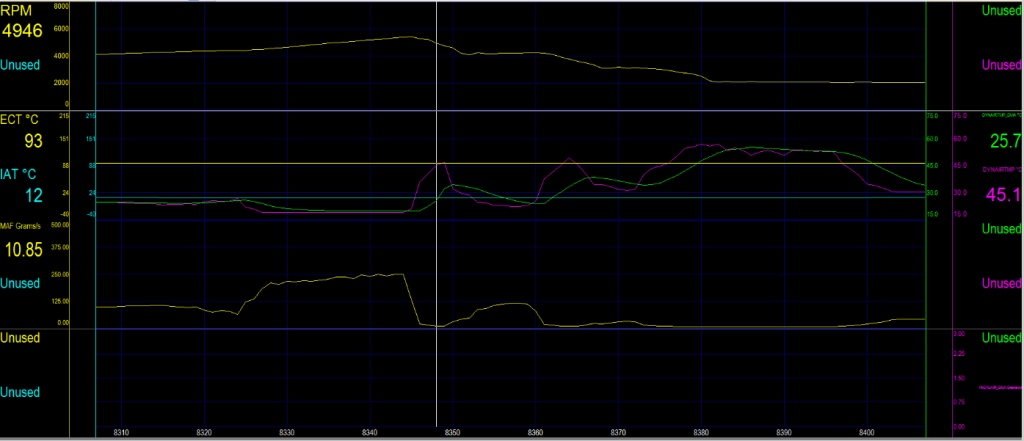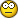1. ##Marcin,

I was a clinician for many years, before retiring from the field. My words were carefully chosen, not in anyway an attack, and based on years of direct experience. Your words cannot hide your underlying mental health issues … get control of yourself and attempt to become a “sociable” member of this Forum … or take a sabbatical until such time as you are able.

Regards,
TazReply With Quote

2. ##awesome, now i'm a mental case for sharing years of my work with complete strangers for free...
Taz, you are correct, i will change. from this moment on, i am taking a sabbatical from helping people.Reply With Quote

3. ##oops. now I've done it.... added to the fray...
I always thought this forum was for the people, by the people, to share ideas?
If one on this board is here to take in what is written, but offers no offerings....
as in... "sharing years of my work with complete strangers for free...,
just why are you here?Reply With Quote

4. ##I don't see anything wrong with what redhardsupra has said.. and I find his post EXTREMELY valuable and...FREE... why would anyone want to piss him off is inexplicable.Reply With Quote

5. ##Originally Posted by redhardsuprawhat's the difference between DYNAIRTMP_DMA vs CHRGTEMP_DMA??
There is no difference. They are the same.Reply With Quote

6. ##Wow!This is a crazy thread!!!!

As a calibrator who has spent years self teaching and mucking through documentation to make my own opinion I have to admit that some things I don't want to share. Not implying to you guys in this thread but many people buy the software...make one post and want the quick answers....I hate that crap. I would rather send info that I guarded through an e mail or PM before I publically dumped it here.

Obviously when discussing general understandings its not that big a deal...

Marcin has done a great job over the years in converting math into street level reality. Much appreciated....maybe if you play nice he will crack! LOL!

HowardReply With Quote

7. ##Originally Posted by redhardsupraCALC.VE is horsepoop. ignore at will.
The CALC.VE pid that comes predefined with the V7 scantool is defined as:

Code:
```VE%   = {SAE.MAF.gps}*({SAE.IAT.C}+273.15)/((displacement()*61.024)*{SAE.RPM}*{SAE.MAP.kPa})*212544
VEpcm = {SAE.MAF.gps}*({SAE.IAT.C}+273.15)/((displacement()*61.024)*{SAE.RPM}*{SAE.MAP.kPa})*6155274.24```
I saw several problems with those definitions:
- they use IAT instead of DAT;
- the constants don't reconcile (VE% is close, VEpcm is nowhere near).

Here's my analysis/derivation of calculated VE (I keep track of the constants):

Code:
```In one cycle (4 strokes, 2 revs) all N cylinders are filled/emptied once.

Time for one cycle (2 revs):
t[s] = 2[rev] * 60[s/min] / RPM[rev/min]
= 120[s*rev/min] / RPM[rev/min]

Mass of air thru engine in one cycle (N cylinders):
VE[g] = MAF[g/s] * t[s]
= MAF[g/s] * 120[s*rev/min] / RPM[rev/min]

Mass of air normalized for temperature and pressure in one cycle (N cylinders):
VE[g*K/kPa] = VE[g] * DAT[K] / MAP[kPa]
= MAF[g/s] * DAT[K] * 120[s*rev/min] / RPM[rev/min] / MAP[kPa]

Normalized mass of air thru one cylinder:
VE[g*K/kPa] = MAF[g/s] * DAT[K] * 120[s*rev/min] / RPM[rev/min] / MAP[kPa] / N
= MAF[g/s] * DAT[K] * 15[s*rev/min] / RPM[rev/min] / MAP[kPa]    when N=8

Ideal Gas Law equation (PV=nRT):
P[Pa] * V[m^3] = n[mol] * R[J/K/mol] * T[K] = (m[g] / M[g/mol]) * R[J/K/mol] * T[K]

where:
M = 28.96[g/mol] = molar mass of air (average molar mass of air composition)
R = 8.31447[J/K/mol] = Universal gas Constant
T = DAT[K]
P = MAP[Pa]
V = displacement[m^3] of engine (all N cylinders)

Theoretical air mass contained in engine displacement V (N cylinders), rearrange IGL:
m[g] = V[m^3] * MAP[Pa] / DAT[K] * M[g/mol] / R[J/K/mol]

Note conversions:
1[Pa] = 1[N/m^2] = 1[J/m^3] since 1[J] = 1[Nm]
1[kPa] = 1000[Pa]
1[m^3] = 1000[L]

So, converting to [L] and [kPa], theoretical air mass becomes:
m[g] = V[L] * MAP[kPa] / DAT[K] * M[g/mol] / R[J/K/mol] * 1000[Pa/kPa] / 1000[L/m^3]
= V[L] * MAP[kPa] / DAT[K] * 28.96[g/mol] / 8.31447[J/K/mol] * 1[Pa/kPa*m^3/L]
= V[L] * MAP[kPa] / DAT[K] * 3.4831[g*K*/kPa/L]

So, mass volumetric efficiency of engine (N cylinders):
VE[%] = VE[g] / m[g] * 100[%]
= MAF[g/s] * 120[s*rev/min] / RPM[rev/min] / V[L] / MAP[kPa] * DAT[K] / 3.4831[g*K*/kPa/L] * 100[%]
= MAF[g/s] * DAT[K] * 3445.2[s/g*kPa/K*rev/min*L*%] / RPM[rev/min] / MAP[kPa] / V[L]

Mass volumetric efficiency of 1 cylinder would be:
VE[%] = (VE[g] / N) / (m[g] / N) * 100[%] = VE[g] / m[g] * 100[%]
= same as for N cylinders.

i.e. VE[%] is the same regardless of number of cylinders.

Writing VE[g*K/kPa] and VE[%] using pids (assuming engine 5.669L V8):
VE[g*K/kPa] = {SAE.MAF.gps}*{CALC.DAT.K}/{SAE.RPM}/{SAE.MAP.kPa}*15  for 1 of 8 cylinders
VE[%]       = {SAE.MAF.gps}*{CALC.DAT.K}/(SAE.RPM}/{SAE.MAP.kPa}*3445.2/5.669```

So I would ignore
the CALC.VE pid (like you said) and instead use the VE derived above.Reply With Quote

8. ##Summary

For one cylinder in an 8 cylinder motor (regardless of displacement):
VE[g*K/kPa] = {SAE.MAF.gps}*{CALC.DAT.K}/{SAE.RPM}/{SAE.MAP.kPa}*15

For a motor having displacement 5.669L (regardless of cylinder count):
VE[%] = {SAE.MAF.gps}*{CALC.DAT.K}/{SAE.RPM}/{SAE.MAP.kPa}*3445.2/5.669Reply With Quote

####Posting Permissions

• You may not post new threads
• You may not post replies
• You may not post attachments
• You may not edit your posts
•